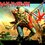# Indeed a general form!!

Solving the last problem made you observe that the first 3 perfect numbers are of the $2^{k-1}(2^{k} - 1)$.

Actually it was proved by Euclid that :

If ($2^{k}-1)$ is a prime the $n = 2^{k-1}(2^{k}-1)$ is perfect and every perfect number of this form.

Try to prove it in the comments box :)

You can also see that this implies all even perfect numbers are triangular numbers.

Also no odd perfect number has been found so far.7 years, 3 months ago

This discussion board is a place to discuss our Daily Challenges and the math and science related to those challenges. Explanations are more than just a solution — they should explain the steps and thinking strategies that you used to obtain the solution. Comments should further the discussion of math and science.

When posting on Brilliant:

• Use the emojis to react to an explanation, whether you're congratulating a job well done , or just really confused .
• Ask specific questions about the challenge or the steps in somebody's explanation. Well-posed questions can add a lot to the discussion, but posting "I don't understand!" doesn't help anyone.
• Try to contribute something new to the discussion, whether it is an extension, generalization or other idea related to the challenge.

MarkdownAppears as
*italics* or _italics_ italics
**bold** or __bold__ bold
- bulleted- list
• bulleted
• list
1. numbered2. list
1. numbered
2. list
Note: you must add a full line of space before and after lists for them to show up correctly
paragraph 1paragraph 2

paragraph 1

paragraph 2

[example link](https://brilliant.org)example link
> This is a quote
This is a quote
    # I indented these lines
# 4 spaces, and now they show
# up as a code block.

print "hello world"
# I indented these lines
# 4 spaces, and now they show
# up as a code block.

print "hello world"
MathAppears as
Remember to wrap math in $$ ... $$ or $ ... $ to ensure proper formatting.
2 \times 3 $2 \times 3$
2^{34} $2^{34}$
a_{i-1} $a_{i-1}$
\frac{2}{3} $\frac{2}{3}$
\sqrt{2} $\sqrt{2}$
\sum_{i=1}^3 $\sum_{i=1}^3$
\sin \theta $\sin \theta$
\boxed{123} $\boxed{123}$

Sort by:

Let $2^k-1=M_p$ [short for Mersenne Prime].

The positive divisors of $n=2^{k-1}(2^k-1)$ are $1$, $2$, $2^2$, $2^3$ $\cdots$ $2^{k-1}$ and ${M_p}, 2M_p, 2^2 M_p, 2^3 M_p, \cdots 2^{k-1} M_p$.

We get $\sigma(n)=2^k-1 + M_p \times (2^k-1)=(2^k-1)(1+M_p)=(2^k-1)\times 2^k=2\times2^{k-1}(2^k-1)=2n$.

So, $n$ is a perfect number by definition.

- 7 years, 3 months ago

When I first found out about perfect numbers (I was 9), I strived to find a pattern between them. After 2 hours only I figured it out but then checked the Internet. Turns out it was discovered by Euclid over 2000 years ago. I still felt proud.

- 7 years, 3 months ago

It was proven by Euler that every even perfect number must have this form.

- 7 years, 3 months ago

I think you're right ..but the general form was given by Euclid right??

- 7 years, 3 months ago

Yes, Euclid found out that $\dfrac{p(p+1)}{2}$ was a perfect number for certain prime numbers $p$. He concluded that those prime numbers had to be of the form $2^k-1$. These primes are now called Mersenne Primes.

Euler later on proved that all even perfect numbers must have this form.

- 7 years, 3 months ago

All even prime numbers?

- 7 years, 3 months ago

Oops! Typo!

- 7 years, 3 months ago